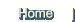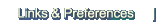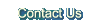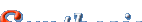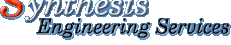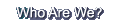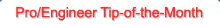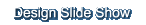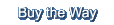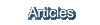<<<   Previous TipPro/E Tips LibraryNext Tip   >>>

Pro/Engineer   April 2005   Tip-of-the-Month

## Power Tools:  Curves by Equation

Our tip this month highlights a very powerful, though less used, function of Pro/E.  As a tool, creating a datum curve from a mathematical equation can be very handy.

Why?  We'll discuss that later.  First, to create a Curve by Equation:

• Pro/E 2001  -  Insert > Datum > Curve … > From Equation > Done > > Select your coordinate system; select Cartesian, Cylindrical or Spherical; then enter your equations.

• Wildfire 2.0  - Insert > Model Datum > Curve … > From Equation > Done > > Select your coordinate system; select Cartesian, Cylindrical or Spherical; then hopefully no bug like mine (I could not edit the equation, it just crashed).  Oh PTC ... why?

Well, it appears functionality is the same in WF2, so back to equations ......

The equations make each of the 3 variables (X, Y, Z in Cartesian Coordinates) independent of each other and based on "t" which Pro/E will vary from 0 to 1.

So, a simple math equation for a parabola, for instance, that is normally written as:

x=y^2
 In Pro/E, an equation must be written for each variable independently like: x=t^2   (x varies with square of t) y=t     (y varies linearly with t) z=0     (curve on the x-y plane)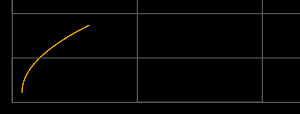Pro/E evaluates the equation from t=0 to t=1, so to scale the result, just add a scale factor to the equations like: x=3*t^2   (scaling the result by 3)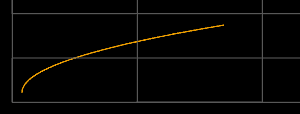Or x=(3*t)^2 Will make the result the same as if  t  varied from 0 to 3.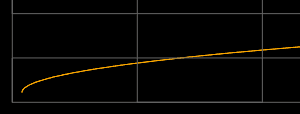Additionally, you can displace the curve by adding a constant to the equation like: x=1.2+3*t^2 And/Or, you can change the Z position by making Z equal to some non Zero value, or even an equation of its own.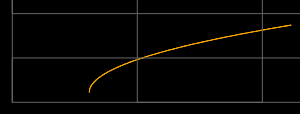That's the simple part.  Now, how do you get control of the equation so you don't have to Redefine it to modify the values?

This requires another feature (or features) for the controlling values -- like a value of another feature -- like a Relation.  Just change the constants in your equations to be parameters defined elsewhere in your model.

TIP:  Create other datum features (like offset planes or curves) then use the dimension values of those to drive your equations.

In the equation example below, a datum plane was created as an offset from a default plane, with a value of 3.  The datum plane offset value has the parameter name d0, so the 3 of the equation  x=3*t^2  is simply replaced with d0 making  x=d0*t^2.

(You can find those parameter names by showing the values (like with Modify) then use Info > Switch Dimensions.  The parameter names show instead of the values.)

Note:  Do not use the & symbol with the parameter names like in equations or drawings.  Why is it different?  Just another inconsistency.

For more control and to really use the available power, expand the above concepts to all the equations.  The example below shows the curve generated from the following equations:

x=d1+d2*t^2
y=d0*t
z=1+d3*cos(t*400)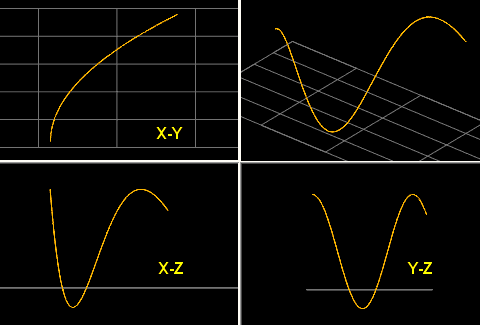Note: that in the X-Y plane, the curve is a parabola, in the Y-Z plane, the curve is a segment of a sine wave, and in the X-Z plane, the curve is a combination.  (The X-Y plane is shown in grid.)

Where do you use equations?  For many things perfect shape is not so important, but for some things, like flow dynamics for instance, it can make a difference.  Some examples:

• For a parabolic curve, most people use sketcher and a conic feature.  Those are great, but if you need to know the values, it is insufficient.

• How can you create a perfectly and smoothly continuous curve in multiple directions?

• Is there another way to insert something that varies as a SIN or COS?
Curves such as these are extremely powerful in creating certain types of geometry, and they are very stable -- assuming, of course, that Pro/E will allow you to create them in the first place.  : )

 By The Way,    (for whomever asked the question),Yes you can, and this is How you create an eliptical helix: x=4*cos(t*3*360) y=2*sin(t*3*360) z=5*t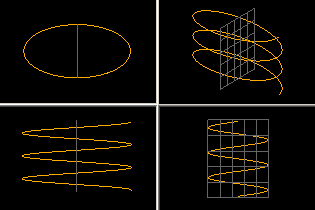We wish you the best of luck using this great Power Tool.This Pro/E Tip-of-the-Month is a service of Synthesis Engineering . . . . . . a Product Development and Engineering Design resources company  . . . . . . Please Contact Us for great Engineering and Development services! Got a Great Tip?   Submit it!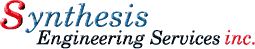Copyright © 1996 -   SYNTHESIS ENGINEERING SERVICES, INC.     :     (719) 380-1122     :     https://www.SYNTHX.com/